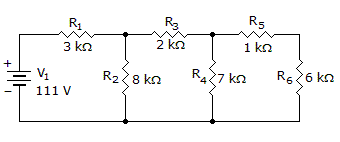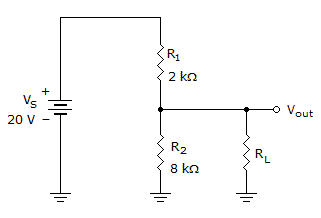# Electronics - Series-Parallel Circuits

Exercise : Series-Parallel Circuits - General Questions
1.
What theorem replaces a complex network with an equivalent circuit containing a source voltage and a series resistance?
Multinetwork
Norton
Thevenin
Superposition
Explanation:
No answer description is available. Let's discuss.

2.
What is the power dissipated by R2, R4, and R6?P2 = 417 mW, P4 = 193 mW, P6 = 166 mW
P2 = 407 mW, P4 = 183 mW, P6 = 156 mW
P2 = 397 mW, P4 = 173 mW, P6 = 146 mW
P2 = 387 mW, P4 = 163 mW, P6 = 136 mW
Explanation:
No answer description is available. Let's discuss.

3.If the load in the given circuit is 120 k, what is the loaded output voltage?

4.21 V
15.79 V
16 V
19.67 V
Explanation:
No answer description is available. Let's discuss.

4.
In a series–parallel circuit, individual component power dissipations are calculated using:
individual component parameters
a percent of the voltage division ratio squared
total current squared multiplied by the resistor values
a percent of the total power depending on resistor ratios